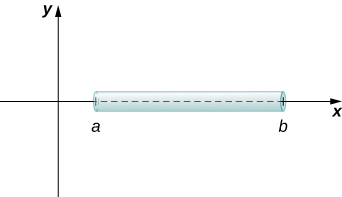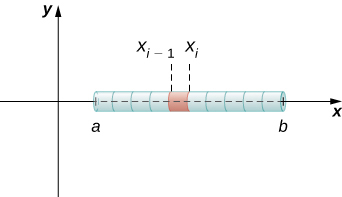# 6.5 Physical applications

 Page 1 / 11
• Determine the mass of a one-dimensional object from its linear density function.
• Determine the mass of a two-dimensional circular object from its radial density function.
• Calculate the work done by a variable force acting along a line.
• Calculate the work done in pumping a liquid from one height to another.
• Find the hydrostatic force against a submerged vertical plate.

In this section, we examine some physical applications of integration. Let’s begin with a look at calculating mass from a density function. We then turn our attention to work, and close the section with a study of hydrostatic force.

## Mass and density

We can use integration to develop a formula for calculating mass based on a density function. First we consider a thin rod or wire. Orient the rod so it aligns with the $x\text{-axis,}$ with the left end of the rod at $x=a$ and the right end of the rod at $x=b$ ( [link] ). Note that although we depict the rod with some thickness in the figures, for mathematical purposes we assume the rod is thin enough to be treated as a one-dimensional object.We can calculate the mass of a thin rod oriented along the x -axis by integrating its density function.

If the rod has constant density $\rho ,$ given in terms of mass per unit length, then the mass of the rod is just the product of the density and the length of the rod: $\left(b-a\right)\rho .$ If the density of the rod is not constant, however, the problem becomes a little more challenging. When the density of the rod varies from point to point, we use a linear density function    , $\rho \left(x\right),$ to denote the density of the rod at any point, $x.$ Let $\rho \left(x\right)$ be an integrable linear density function. Now, for $i=0,1,2\text{,…},n$ let $P=\left\{{x}_{i}\right\}$ be a regular partition of the interval $\left[a,b\right],$ and for $i=1,2\text{,…},n$ choose an arbitrary point ${x}_{i}^{*}\in \left[{x}_{i-1},{x}_{i}\right].$ [link] shows a representative segment of the rod.A representative segment of the rod.

The mass ${m}_{i}$ of the segment of the rod from ${x}_{i-1}$ to ${x}_{i}$ is approximated by

${m}_{i}\approx \rho \left({x}_{i}^{*}\right)\left({x}_{i}-{x}_{i-1}\right)=\rho \left({x}_{i}^{*}\right)\text{Δ}x.$

Adding the masses of all the segments gives us an approximation for the mass of the entire rod:

$m=\sum _{i=1}^{n}{m}_{i}\approx \sum _{i=1}^{n}\rho \left({x}_{i}^{*}\right)\text{Δ}x.$

This is a Riemann sum. Taking the limit as $n\to \infty ,$ we get an expression for the exact mass of the rod:

$m=\underset{n\to \infty }{\text{lim}}\sum _{i=1}^{n}\rho \left({x}_{i}^{*}\right)\text{Δ}x={\int }_{a}^{b}\rho \left(x\right)dx.$

We state this result in the following theorem.

## Mass–density formula of a one-dimensional object

Given a thin rod oriented along the $x\text{-axis}$ over the interval $\left[a,b\right],$ let $\rho \left(x\right)$ denote a linear density function giving the density of the rod at a point x in the interval. Then the mass of the rod is given by

$m={\int }_{a}^{b}\rho \left(x\right)dx.$

We apply this theorem in the next example.

## Calculating mass from linear density

Consider a thin rod oriented on the x -axis over the interval $\left[\pi \text{/}2,\pi \right].$ If the density of the rod is given by $\rho \left(x\right)=\text{sin}\phantom{\rule{0.2em}{0ex}}x,$ what is the mass of the rod?

Applying [link] directly, we have

$m={\int }_{a}^{b}\rho \left(x\right)dx={\int }_{\pi \text{/}2}^{\pi }\text{sin}\phantom{\rule{0.2em}{0ex}}x\phantom{\rule{0.2em}{0ex}}dx={\text{−}\text{cos}\phantom{\rule{0.2em}{0ex}}x|}_{\pi \text{/}2}^{\pi }=1.$

Consider a thin rod oriented on the x -axis over the interval $\left[1,3\right].$ If the density of the rod is given by $\rho \left(x\right)=2{x}^{2}+3,$ what is the mass of the rod?

$70\text{/}3$

We now extend this concept to find the mass of a two-dimensional disk of radius $r.$ As with the rod we looked at in the one-dimensional case, here we assume the disk is thin enough that, for mathematical purposes, we can treat it as a two-dimensional object. We assume the density is given in terms of mass per unit area (called area density ), and further assume the density varies only along the disk’s radius (called radial density ). We orient the disk in the $xy\text{-plane,}$ with the center at the origin. Then, the density of the disk can be treated as a function of $x,$ denoted $\rho \left(x\right).$ We assume $\rho \left(x\right)$ is integrable. Because density is a function of $x,$ we partition the interval from $\left[0,r\right]$ along the $x\text{-axis}.$ For $i=0,1,2\text{,…},n,$ let $P=\left\{{x}_{i}\right\}$ be a regular partition of the interval $\left[0,r\right],$ and for $i=1,2\text{,…},n,$ choose an arbitrary point ${x}_{i}^{*}\in \left[{x}_{i-1},{x}_{i}\right].$ Now, use the partition to break up the disk into thin (two-dimensional) washers. A disk and a representative washer are depicted in the following figure.

#### Questions & Answers

Find the derivative of g(x)=−3.
Abdullah Reply
any genius online ? I need help!!
Guzorochi Reply
how can i help you?
Pina
need to learn polynomial
Zakariya
i will teach...
nandu
I'm waiting
Zakariya
plz help me in question
Abish
evaluate the following computation (x³-8/x-2)
Murtala Reply
teach me how to solve the first law of calculus.
Uncle Reply
what is differentiation
Ibrahim Reply
f(x) = x-2 g(x) = 3x + 5 fog(x)? f(x)/g(x)
Naufal Reply
fog(x)= f(g(x)) = x-2 = 3x+5-2 = 3x+3 f(x)/g(x)= x-2/3x+5
diron
pweding paturo nsa calculus?
jimmy
how to use fundamental theorem to solve exponential
JULIA Reply
find the bounded area of the parabola y^2=4x and y=16x
Omar Reply
what is absolute value means?
Geo Reply
Chicken nuggets
Hugh
🐔
MM
🐔🦃 nuggets
MM
(mathematics) For a complex number a+bi, the principal square root of the sum of the squares of its real and imaginary parts, √a2+b2 . Denoted by | |. The absolute value |x| of a real number x is √x2 , which is equal to x if x is non-negative, and −x if x is negative.
Ismael
find integration of loge x
Game Reply
find the volume of a solid about the y-axis, x=0, x=1, y=0, y=7+x^3
Godwin Reply
how does this work
Brad Reply
Can calculus give the answers as same as other methods give in basic classes while solving the numericals?
Cosmos Reply
log tan (x/4+x/2)
Rohan
please answer
Rohan
y=(x^2 + 3x).(eipix)
Claudia
is this a answer
Ismael
A Function F(X)=Sinx+cosx is odd or even?
WIZARD Reply
neither
David
Neither
Lovuyiso
f(x)=1/1+x^2 |=[-3,1]
Yuliana Reply
apa itu?
fauzi

### Read also:

#### Get Jobilize Job Search Mobile App in your pocket Now!

Source:  OpenStax, Calculus volume 1. OpenStax CNX. Feb 05, 2016 Download for free at http://cnx.org/content/col11964/1.2
Google Play and the Google Play logo are trademarks of Google Inc.

Notification Switch

Would you like to follow the 'Calculus volume 1' conversation and receive update notifications?By OpenStaxBy OpenStaxBy OpenStaxBy OpenStaxBy OpenStaxByBy OpenStaxBy David CoreyBy Robert MurphyBy Szilárd Jankó